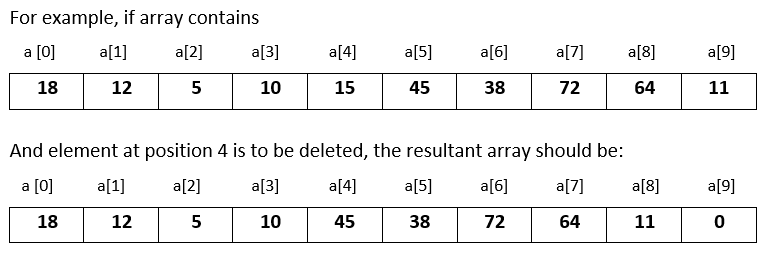# Input 10 numbers in 1d array and delete the number at the given position in Java

###### One Dimensional Array - Question 9

In this question, we will see how to input 10 numbers in a one dimensional integer array and delete a number from the array at a given position in Java programming. To know more about one dimensional array click on the one dimensional array lesson.

Q9) Write a program in Java to input 10 numbers in a one dimensional integer array and input a position. Now delete the number at that position by shifting the rest of the numbers to the left and insert a 0 at the end.#### Program

``````import java.util.Scanner;

public class Q9
{
public static void main(String args[])
{
int a[]=new int, i,p;
Scanner sc=new Scanner(System.in);

System.out.println("Enter 10 numbers");
for(i=0; i<10; i++)
{
a[i]=sc.nextInt();
}

System.out.print("Enter position to delete the number ");
p=sc.nextInt();
for(i=p; i<9; i++)
{
a[i]=a[i+1];
}
a[i]=0;

System.out.println("\nModified array after deleting the number");
for(i=0; i<10; i++)
{
System.out.print(a[i]+" ");
}
}
}``````

#### Output

```Enter 10 numbers
18
12
5
10
15
45
38
72
64
11
Enter position to delete the number 4

Modified array after deleting the number
18 12 5 10 45 38 72 64 11 0                ```